http://www.dmst.aueb.gr/dds/pubs/conf/1999-Perf-GASA/html/gasa.html This is an HTML rendering of a working paper draft that led to a publication. The publication should always be cited in preference to this draft using the following reference: Diomidis Spinellis and Chrissoleon T. Papadopoulos. Production line buffer allocation: Genetic algorithms versus simulated annealing. In Second International Aegean Conference on the Analysis and Modelling of Manufacturing Systems, pages 89–101. University of the Aegean, Department of Business Administration, May 1999.Citation(s): 7 (selected).This document is also available in PDF format.The document's metadata is available in BibTeX format. This material is presented to ensure timely dissemination of scholarly and technical work. Copyright and all rights therein are retained by authors or by other copyright holders. All persons copying this information are expected to adhere to the terms and constraints invoked by each author's copyright. In most cases, these works may not be reposted without the explicit permission of the copyright holder.

PRODUCTION LINE BUFFER ALLOCATION:
GENETIC ALGORITHMS VERSUS SIMULATED ANNEALING

 Diomidis D. Spinellis Chrissoleon T. Papadopoulos Department of Mathematics Department of Business Administration GR-832 00 Karlovassi GR-821 00 Chios University of the Aegean University of the Aegean Greece Greece dspin@aegean.gr hpap@aegean.gr

We present and compare two stochastic approaches for solving the buffer allocation problem in reliable production lines. The problem entails the determination of near optimal buffer allocation plans in large production lines with the objective of maximizing their throughput. The allocation plan is calculated subject to a given amount of total buffer slots using simulated annealing and genetic algorithms. The throughput is calculated utilizing a decomposition method.

Keywords: Simulated annealing, genetic algorithms, production lines, buffer allocation, decomposition method

# 1 Introduction and Literature Review

The allocation of buffers between workstations is a major optimization problem faced by manufacturing systems designers. It has to do with devising an allocation plan for distributing a certain amount of buffer space among the intermediate buffers of a production line. It is a very complex task that must account for the random fluctuations in mean production rates of the individual workstations of the lines. To solve this problem there is a need for two different tools. The first is a tool that calculates the performance measure of the line which has to be optimized (e.g., the throughput or the mean work-in-process). This may be an evaluative method such as simulation, a decomposition method [Ger87,DF93], or a traditional Markovian state model in conjunction with an exact numerical algorithm like [HPB93]. The second tool is a search (generative) method that tries to determine an optimal or near optimal value for the decision variables, which in our case are the buffer capacities of the intermediate buffer locations in the line. Examples of such methods are the classical search methods such as the well-known Hooke-Jeeves method, various heuristic methods, knowledge based methods, genetic algorithms, and simulated annealing.

Evaluative and generative (optimization) models can be combined in a closed loop' configuration by using feedback from an evaluative model to modify the decision taken by the generative model. In such a configuration the evaluative model is used to obtain the value of the objective function for a set of inputs. The value of the objective function is then communicated to the generative model which uses it as an objective criterion in its search for an optimal solution. In the rest of this paper we will use the formalism $S(G, E)$ to describe a closed loop system using the generative method $G$ and the evaluative method $E$. The generative models that will be used in this paper are:

CE
complete enumeration,
RE
reduced enumeration,
GA
genetic algorithms, and
SA
simulated annealing.
Furthermore, two evaluative models will be used:
Exact
the exact numerical algorithm [HPB93], and
Deco
the decomposition algorithm numbered as A3 in [DF93].

For a systematic review of the existing literature in the area of evaluative and generative models of manufacturing systems, the interested reader is addressed, respectively, to two review papers by [DG92] and [PH96] and to the books by [PHB93], [AS93], [BS93], [Ger94], [Per94] and [Alt97], among others.

Several researchers have studied the problem of optimizing buffer allocation to maximize the efficiency of a reliable production line, (see for example, [HS91], and [HSB93]). These methods are based on comprehensive studies to characterize the optimal buffer allocation pattern. Authors have provided extensive numerical results for balanced lines with up to 6 stations and limited results for lines with up to 9 stations. However, few methods can handle this problem for large production lines, in a computationally efficient way. In this paper we compare two stochastic approaches suitable for large production lines, one based on genetic algoriths and one based on simulated annealing. Details on how these methods can be applied to the problem are given in [BDI95] which describes the application of genetic algorithms for the buffer allocation in asynchronous assembly systems and in [SP99b] for a corresponding approach using simulated annealing. The implementation of both approaches in this paper works in close cooperation with a decomposition method as given in [DF93].

Simulated annealing is an adaptation of the simulation of physical thermodynamic annealing principles described by [MRR+53] to the combinatorial optimization problems [KJV83,Cer85]. Similar to genetic algorithms and tabu search techniques [Glo90] it follows the local improvement'' paradigm for harnessing the exponential complexity of the solution space. The algorithm is based on randomization techniques. An overview of algorithms based on such techniques can be found in [GSB94]. A complete presentation of the method and its applications can be found in [LA87] and accessible algorithms for its implementation are presented by [CMMR87,PFTV88]. As a tool for operational research simulated annealing is presented by [Egl90], while [KAJ94] provide a complete survey of simulated annealing applications to operations research problems.

Genetic algorithms [Hol75,Gol89,For96] are global optimization techniques that avoid many of the shortcomings exhibited by local search techniques on difficult search spaces, such as the buffer allocation problem. Genetic algorithm applications are given in [Gol94], their use for modelling, design, and process control is presented in [Kar93], while the methodology used for optimizing simulated systems can be found in [TA95].

This paper is organized as follows. Section 2 states the problem and the assumptions of the model, whereas, Section 3 describes the evaluation methodology and associated implementation decisions. In Section 4, we compare the numerical results obtained from the algorithms. Finally, Section 5 concludes the paper and suggests some future research directions.

# 2 Assumptions of the Model and the Buffer Allocation Problem

In asynchronous production lines, each part enters the system from the first station, passes in order from all stations and the intermediate buffer locations and exits the line from the last station. The flow of the parts works as follows: in case a station has completed its processing and the next buffer has space available, the processed part is passed on. Then, the station starts processing a new part that is taken from its input buffer. In case the buffer has no parts, the station remains empty until a new part is placed in the buffer. This type of line is subject to manufacturing blocking (or blocking after service) and starving.

Assumptions of the model: It is assumed that the first station is never starved and the last station is never blocked. The processing (service) times at each station are assumed to be independent random variables following the exponential distribution, with mean service rates, $μ$i , $i=1,2,&ldots;, K$. In our model, the stations of the line are assumed to be perfectly reliable, that is, breakdowns are not allowed.

The exponentiality of the processing times as well as the absolute reliability of the line's workstations are rather unrealistic assumptions. However, the service completion times can be exponential or can be approximated by an exponential distribution. The variability in completion times may be attributed to failures and repairs which implicitly exist in the problem at hand. Following this view, the proposed model may be applied to any unreliable production line under the exponentiality assumptions for the service completion times.

Figure 1 depicts a $K$-station line that has $K-1$ intermediate locations for buffers, labelled $B$2, B3, &ldots;, BK .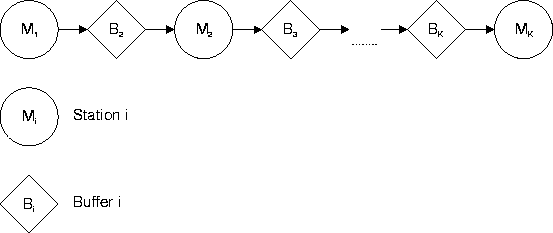The basic performance measures in the analysis of production lines are the throughput (or mean production rate) and the average work-in-process (WIP) or equivalently the average production (sojourn) time.

The object of the present work is the buffering of asynchronous, reliable production lines with the assumptions given above. The objective is the maximization of the line's throughput, subject to a given total buffer space.

The buffer allocation problem: In mathematical terms, our problem can be stated as follows:

P
Find B=(B2,B3,&ldots;,BK) so as to

  maxOK(B) (1)
subject to:(2)
where: $N$ is a fixed nonnegative integer, denoting the total buffer space available in the production line.

B=(B2,B3,&ldots;,BK) is the buffer vector', i.e., a vector with elements the buffer capacities of the $K-1$ buffers.

$O$K , denotes the throughput of the $K$-station line. This is a function of the mean service rates of the $K$ stations, $μ$i,   (i=1,2,&ldots;,K) , of the coefficients of variation, $CV$i , of the service times and the buffer capacities, $B$i .

Methodology of investigation: To evaluate approaches for solving the optimal buffer allocation problem (P) for large production lines, we have performed the following steps:

S1
We utilized the decomposition method given by [DF93], as an evaluative tool, to determine the throughput of the lines. The algorithm computes approximately the throughput for any $K$-station line with finite intermediate buffers and exponentially distributed processing times. The number of feasible allocations of $N$ buffer slots among the $K-1$ intermediate buffer locations increases dramatically with $N$ and $K$ and is given by the formula: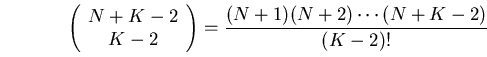(3)

S2
To find the buffer allocation that maximizes the throughput of the line, we utilized a simulated annealing and a genetic algorithm method specifically adapted for solving this problem. Our approach is described in the following section.

# 3 Evaluation Methodology

In order to evaluate the applicability of the stochastic methods to the buffer allocation problem we designed and implemented a system to calculate the optimum buffer configuration for a given reliable production line using a variety of algorithms [SP99a]. The system takes as input:

• the number of stations in the production line, $K$,
• the available buffer space, $N$, and
• the station mean service rates, $μ$i , $i=1,2,&ldots;, K$.
Based on the above input, the system calculates the buffer allocations B=(B2,B3,&ldots;,BK) for the maximal line throughput. Furthermore, the system is instrumented to provide as part of the solution the throughput of the suggested configuration, as well as the number of different configurations that were tried. The line throughput is used to evaluate the quality of the suggested configuration when compared with the throughput calculated by other methods. The number of different configurations tried, is used as an objective performance criterion, because the configuration evaluation step is the dominant execution time factor and the basic building block of all optimization methods.

We ran a number of tests on both balanced and unbalanced lines and compared the simulated annealing results against the results obtained by other methods. For short lines and limited buffer space a complete enumeration of all configurations provided an accurate measure when comparing with the simulated annealing results. For larger configurations we used a reduced enumeration in order to provide the comparative measure.

Reduced enumeration is based on the experimental observation that the absolute difference of the respective elements of the optimal buffer allocation (OBA) vectors with $N$ and $N+1$ buffer slots is less than or equal to 1:

$|B$iN+1 - BiN| ≤1,   ∀i: 2 ≤i ≤K.

In this way, we have been able to derive the OBA by induction for any number $N$ of buffer slots that are to be allocated among the $K-1$ buffer locations of the line. The reduction works as follows: when $N*$ and $K$ are given one needs to determine all the OBA vectors for $N=1,2,&ldots;,N*$ and then for $N=N*+1$ by searching only the values of $B$iN-1 , $B$iN and $B$iN+1 . Furthermore, this reduction starts after a number of total buffer slots $N$. To quantify the reduction, by applying the improved enumeration it has been experimentally observed that the number of iterations were reduced by at least 60% for short lines. This reduction accounts for well over 90% for large production lines (with more than 12 stations). Recall that the number of feasible allocations of $N$ buffer slots among the $K-1$ intermediate buffer locations increases dramatically with $N$ and $K$ and is given by formula (3).

Simulated annealing is an optimization method suitable for combinatorial minimization problems. Such problems exhibit a discrete, factorially large, configuration space. In common with all paradigms based on local improvements'' the simulated annealing method starts with a non-optimal initial configuration (which may be chosen at random) and works on improving it by selecting a new configuration using a suitable mechanism (at random in the simulated annealing case) and calculating the corresponding cost differential ( $ΔO$K ). If the cost is reduced, then the new configuration is accepted and the process repeats until a termination criterion is satisfied. Unfortunately, such methods can become trapped'' in a local optimum that is far from the global optimum. Simulated annealing avoids this problem by allowing uphill'' moves based on a model of the annealing process in the physical world.

Our implementation of the simulated annealing algorithm for distributing $N$ buffer space in a $K$-station line -- described in detail in [SP99b] -- follows the following steps:

1.
$[$Set initial line configuration.$]$ Set $B$i ←⌊N/K ⌋ , set $B$K/2 ←BK/2 + N - ∑i=2K ⌊N/K ⌋ .
2.
$[$Set initial temperature $T$0 .$]$ Set $T ←0.5$.
3.
$[$Initialize step and success count.$]$ Set $S ←0$, set $U ←0$.
4.
$[$Create new line with a random redistribution of buffer space.$]$ Move $R$n space from a source buffer $B$Rs to a destination buffer $B$Rd : set B' ←B , set $R$s ←⌊rand [2 &ldots;K+1)⌋ , set $R$d ←⌊rand [2 &ldots;K+1)⌋ , set $R$n ←⌊rand [0 &ldots;BRs+1)⌋ , set $B$Rs ←BRs - Rn , set $B$Rd ←BRd + Rn .
5.
$[$Calculate energy differential.$]$ Set $ΔE ← O$K(B) - OK(B') .
6.
$[$Decide acceptance of new configuration.$]$ Accept all new configurations that are more efficient and, following the Boltzmann probability distribution, some that are less efficient: if $ΔE < 0$ or $exp({-ΔET}) > rand (0 &ldots;1)$, set BB' , set $U ←U + 1$.
7.
$[$Repeat for current temperature$]$. Set $S ←S + 1$. If $S < maximum number of steps$, go to step 4.
8.
$[$Lower the annealing temperature.$]$ Set $T ←c T$ ($0 < c < 1$).
9.
$[$Check if progress has been made.$]$ If $U > 0$, go to step 3; otherwise the algorithm terminates.

Genetic algorithms are also global optimization techniques that avoid many of the shortcomings exhibited by local search techniques on difficult search spaces. They rely on modelling the problem as a population of organisms. Every organism represents a possible valid solution to the problem. Organisms are composed of alleles representing parts of a given solution. Standard genetic recombination operators are used to create new organisms out of existing ones by combining alleles of the existing organisms. In addition, mutations can randomly change the composition of existing organisms. Typically, the algorithm evaluates all the organisms of the population and creates new organisms by combining existing ones based on their fitness. This procedure is repeated until the variance of the population reaches a predefined minimum value.

An important characteristic of our implementation of the genetic algorithm concerns the representation of the solution. A good representation should ensure that the application of standard crossover recombination operators (where a new organism is composed from parts of two existing ones) would result in a valid new representation. Representing the line configuration as a vector $B$ of buffers allocated across the line is not such a representation since given two buffer configurations $($B1, B2) and recombining them as a new buffer B' at point $c$ so that $B'$0 &ldots;c ←B1, 0 &ldots;c and $B'$c + 1 &ldots;K ←B2, c + 1 &ldots;K will not guarantee that $∑$i=1KB'i = N i.e. that the resulting line configuration will be composed of $N$ buffers. For this reason we devised an alternative, position-based, representation using a vector P of length equal to the number of buffers $N$. Every element of P can take values $0 &ldots;K$ representing the position of the given buffer slot within the production line. The two representations are equivalent; the vector P can be mapped to B as follows: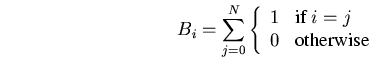(4)
The position-based representation will generate valid buffer configurations using standard genetic crossover and mutation operators. Using this representation, the genetic algorithm we implemented for distributing $N$ buffer space in a $K$-station line is described in the following steps:

1.
$[$Initialize a population of size $S$.$]$ Set $P$0 &ldots;S,0 &ldots;N ←⌊rand [0 &ldots;K-1)⌋ .
2.
$[$Evaluate population members creating throughput vector T .$]$ For $i ←0 &ldots;S$: set $T$i ←OK(Pi) .
3.
$[$Create roulette selection probability vector R . $]$ Set $R$i ←∑j=0i (Tj / ∑k=0STk) .
4.
$[$Create new population using crossovers from the previous population.$]$ For $i ←0 &ldots;S$: if $rand [0 &ldots;1) < crossover rate$, set $c ←⌊rand [0 &ldots;S)⌋$, set $P'$i, 0 &ldots;c ← PRr, 0 &ldots;c , set $P'$i, c + 1 &ldots;N ← PRr, c + 1 &ldots;N ; otherwise set P'iPRr by selecting each $r$ using the roulette selection probability vector so that $R$r ≤rand [0 &ldots;1) < Rr+1
5.
$[$Introduce mutations.$]$ For $i ←0 &ldots;S$: for $j ←0 &ldots;N$: if $rand [0 &ldots;1) < mutation rate$, set $P'$i,j ←⌊rand [0 &ldots;K-1)⌋ .

6.
$[$Keep fittest organism for elitist selection strategy.$]$ Select $f$ so that $T$f ≥T0 &ldots;S , set P'⌊rand [0 &ldots;S)⌋Pf
7.
$[$Make new population the current population.$]$ Set PP' .
8.
$[$Loop based on the population's variance.$]$ If $∑$i=0P | Tf - Ti | > maximum variance go to step 2; otherwise the algorithm terminates with the optimal line setup in Pf .

The implementation of genetic algorithms can be tuned using a number of different parameters. In our implementation we used the parameters presented in [Gre86], namely a population size $S$ of $50$, a crossover rate of $0.6$, a mutation rate of $0.0001$, a generation gap of 1 (the entire population is replaced during each generation), no scaling window, and an elitist selection strategy (the organism with the best performance survives intact into the next generation).

The random floating point numbers $0 < R < 1$ used for selecting energy differentials based on the annealing temperature $R < exp({-ΔET})$, the crossover points, the mutation rates, and the selection of organisms are produced using the subtractive method algorithm described in [Knu81]. Finally, the evaluative function that we used for calculating $ΔE$ is based on the decomposition method [DF93].

# 4 Method comparison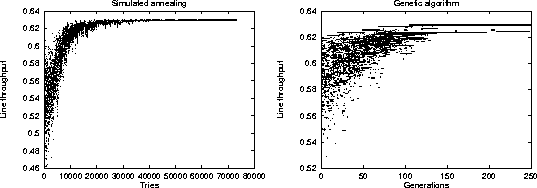Before detailing the comparative results of our examination, it is interesting to visualize the operation of the two stochastic methods. Figure 2 depicts the runtime behaviour of the two methods. Each point on the two scatter charts represents a given line throughput value at a specific step of the algorithm. Both charts depict the calculation of the placement of 30 buffers in a balanced line of 15 stations. The simulated annealing algorithm optimizes a single solution in the specific example in $80.000$ iterations. The solution's throughput value oscillates as both better and worse solutions are randomly selected at each iteration step. As can be seen on the chart, the oscillation width decreases following the algorithm's exponential cooling schedule and converges towards the optimal value.

In contrast to the simulated annealing algorithm, the genetic algorithm is based on the implicit parallelism of the solutions represented by the initial population. Thus, in the specific example, it terminates with an optimal configuration after 250 generations. As the chart demonstrates the search starts with a wide spectrum of different solutions which are evaluated and evolve in parallel with non-optimal solutions gradually becoming extinct. Mutations and recombinations regenerate suboptimal solutions, but, due to the probabilistic organism selection strategy, their survival does not last for long.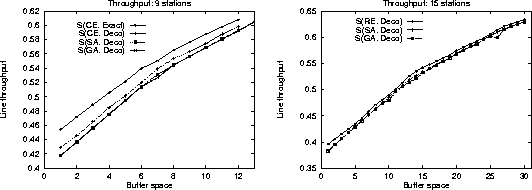Our first comparison experiment concerned the algorithm operation on balanced lines for cases where exact solutions were known. In Figure 3 we present the optimum throughput configurations for balanced lines found using the stochastic methods against the throughput found using complete (for 9 stations) and reduced enumeration techniques. It is apparent that the stochastic algorithm results are almost identical and follow closely the results obtained by the other methods. Both methods are subject to the reduced evaluative accuracy of the decomposition method compared to the Markovian model.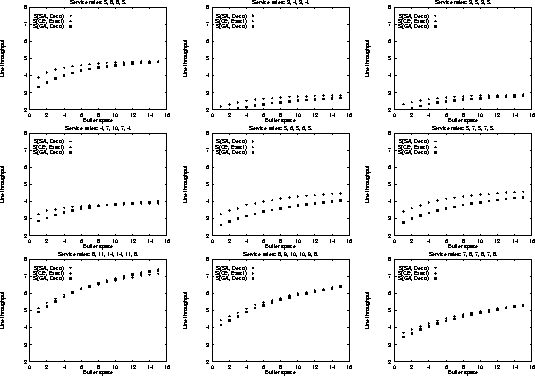In addition to the balanced line evaluation, we compared the stochastic methods against unbalanced line enumeration using the Markovian evaluative procedure for a variety of line sizes, service time configurations, and available buffer space. The results are summarized in Figure 4. It is apparent, that the stochastic method configurations -- although identical to each other -- are not always optimal for limited available buffer space; however, they quickly converge towards the optimal configurations as buffer space increases. This difference can be accounted by the use of the fast decomposition evaluative procedure used in the stochastic algorithm implementation yielding approximate results against the use of the Markovian evaluative procedure for the enumeration method yielding exact results.The goal for using stochastic methods is to optimize large production line problems where the cost of other methods is prohibitevely expensive. As an example the reduced enumeration method when run on a 15 station line with a buffer capacity of 30 units took more than 10 hours to complete on a 100MHz Pentium processor. As shown in Figure 5 the cost of the stochastic methods is higher than the cost of the full and reduced enumeration methods for small lines and buffer allocations. However, it quickly becomes competitive as the number of stations and the available buffer size increase. In addition, the performance of the genetic algorithm implementation is approximately an order of magnitude better than the simulated annealing implementation. Notice that -- in contrast to the deterministic methods -- the stochastic method cost does not increase together with the available buffer space and that it increases only linearly with the number of stations.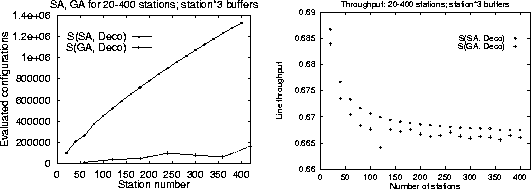Finally, Figure 6 depicts the comparative performance and calculated throughput for the two stochastic methods when optimizing lines of up to $400$ stations and $1200$ buffers. The genetic algorithm implementation producing solutions with only $2.000.000$ evaluations even for $400$ station lines is clearly the performance winner. However, as depicted on the right hand chart, the throughput of the line configuration found by the genetic algorithm is consistently lower than the throughput of the line found by the simulated annealing method. The results we obtained could not be independently verified, because no other numerical results for the buffer allocation problem in large production lines can be found in the open literature.

# 5 Conclusions and Further Work

The results obtained applying stochastic methods to the reliable line near-optimal buffer allocation problem are interesting. The performance and the accuracy of the methods, although inferior for optimizing small lines with limited buffer space, indicate clearly that they become the methods of choice as the problem size increases. Both methods can be used for optimizing large line configurations with simulated annealing producing more optimal configurations and the genetic algorithm approach leading in performance. This indicates that the two methods can be used in complimentary fashion. Real-time applications can utilise genetic algorithms for the swift recalculation of optimal configurations, while batch-oriented calculations can utilise simulated annealing for obtaining an optimal configuration.

Further investigation is needed in order to fully evaluate the potential of the two methods. The failure, in large production lines, of the genetic algorithm method to locate the optimal configuration found by the simulated annealing method is intriguing. It would be interesting to carefully examine the endgames'' of the two methods and find if and how the genetic algorithm implementation can be tweaked to evolve towards more optimal configurations. A dynamic re-adjustment of the algorithm's parameters (population size, crossover rate, mutation rate, etc.) forms one such possibility.

The annealing schedule and the genetic algorithm parameters that we used can clearly be optimized potentially increasing both methods' accuracy and performance. The use of heuristics in setting up the initial buffer configuration can decrease the number of steps needed for reaching the optimal. Finally, we would like to test and compare the methods' potential on similar problems especially involving parallel station production lines.

$#1$

## References

Alt97
T. Altiok.
Performance Analysis of Manufacturing Systems.
Springer-Verlag, New York, 1997.

AS93
R. G. Askin and C. R. Standridge.
Modeling and Analysis of Manufacturing Systems.
John Wiley, New York, 1993.

BDI95
A. A. Bulgak, P. D. Diwan, and B. Inozu.
Buffer size optimization in asynchronous assembly systems using genetic algorithms.
Computers ind. Engng, 28(2):309-322, 1995.

BS93
J. A. Buzacott and J. G. Shanthikumar.
Stochastic Models of Manufacturing Systems.
Prentice Hall, New Jersey, 1993.

Cer85
V. Cerny.
Thermodynamical approach to the traveling salesman problem: an efficient simulation algorithm.
Journal of Optimization Theory and Applications, 45:41-51, 1985.

CMMR87
A. Corana, M. Marchesi, C. Martini, and S. Ridella.
Minimizing multimodal functions of continuous variables with the simulated annealing'' algorithm.
ACM Transactions on Mathematical Software, 13(3):262-280, September 1987.

DF93
Y. Dallery and Y. Frein.
On decomposition methods for tandem queueing networks with blocking.
Operations Research, 41(2):386-399, 1993.

DG92
Y. Dallery and S. B. Gershwin.
Manufacturing flow line systems: A review of models and analytical results.
Queueing Systems: Theory and Applications, 12:3-94, 1992.

Egl90
R. W. Eglese.
Simulated annealing: A tool for operational research.
European Journal of Operational Research, 46:271-281, 1990.

For96
Stephanie Forrest.
Genetic algorithms.
ACM Computing Surveys, 28(1):77-83, March 1996.

Ger87
S. B. Gershwin.
An efficient decomposition method for the approximate evaluation of tandem queues with finite storage space and blocking.
Operations Research, 35(2):291-305, 1987.

Ger94
S. B. Gershwin.
Manufacturing Systems Engineering.
Prentice Hall, New Jersey, 1994.

Glo90
F. Glover.
Tabu search -- part I.
ORSA Journal on Computing, 1:190-206, 1990.

Gol89
David E. Goldberg.
Genetic Algorithms: In Search of Optimization & Machine Learning.

Gol94
David E. Goldberg.
Genetic and evolutionary algorithms come of age.
Communications of the ACM, 37(3):113-119, March 1994.

Gre86
John J. Grefenstette.
Optimization of control parameters for genetic algorithms.
IEEE Transactions on Systems, Man, and Cybernetics, 16(1):122-128, 1986.

GSB94
R. Gupta, S. A. Smolka, and S. Bhaskar.
On randomization in sequential and distributed algorithms.
ACM Computing Surveys, 26(1):7-86, 1994.

Hol75
J. H. Holland.
Adaptation in Natural and Artificial Systems.
University of Michigan Press, Ann Arbor, Michigan, 1975.

HPB93
C. Heavey, H. T. Papadopoulos, and J. Browne.
The throughput rate of multistation unreliable production lines.
European Journal of Operational Research, 68:69-89, 1993.

HS91
F. S. Hillier and K. C. So.
The effect of the coefficient of variation of operation times on the allocation of storage space in production line systems.
IIE Transactions, 23(2):198-206, 1991.

HSB93
F. S. Hillier, K. C. So, and R. W. Boling.
Notes: Toward characterizing the optimal allocation of storage space in production line systems with variable processing times.
Management Science, 39(1):126-133, 1993.

KAJ94
C. Koulamas, S. R. Antony, and R. Jaen.
A survey of simulated annealing applications to operations research problems.
Omega International Journal of Management Science, 22(1):41-56, 1994.

Kar93
Charles L. Karr.
Genetic algorithms for modelling, design, and process control.
In CIKM '93. Proceedings of the second international conference on Information and knowledge management, pages 233-238. ACM, 1993.

KJV83
S. Kirkpatrick, C. D. Gelatt Jr., and M. P. Vecchi.
Optimization by simulated annealing.
Science, 220:671-679, 1983.

Knu81
D. E. Knuth.
The Art of Computer Programming, volume 2 / Seminumerical Algorithms, pages 171-173.

LA87
P. J. M. Van Laarhoven and E. H. L. Aarts.
Simulated Annealing: Theory and Applications.
D. Reidel, Dordrecht, The Nethelands, 1987.

MRR+53
N. Metropolis, A. N. Rosenbluth, M. N. Rosenbluth, A. H. Teller, and E. Teller.
Equation of state calculation by fast computing machines.
Journal of Chemical Physics, 21(6):1087-1092, 1953.

Per94
H. Perros.
Queueing Networks with Blocking.
Oxford University Press, 1994.

PFTV88
W. H. Press, B. P. Flannery, S. A. Teukolsky, and W. T. Vetterling.
Numerical Recipes in C, pages 343-352.
Cambridge University Press, 1988.

PH96
H. T. Papadopoulos and C. Heavey.
Queueing theory in manufacturing systems analysis and design: A classification of models for production and transfer lines.
European Journal of Operational Research, 92:1-27, 1996.

PHB93
H. T. Papadopoulos, C. Heavey, and J. Browne.
Queueing Theory in Manufacturing Systems Analysis and Design.
Chapman and Hall, London, 1993.

SP99a
Diomidis Spinellis and Chrissoleon T. Papadopoulos.
ExPLOre: A modular architecture for production line optimisation.
In Proceedings of the 5th International Conference of the Decision Sciences Institute, Athens, Greece, July 1999.
To appear.

SP99b
Diomidis Spinellis and Chrissoleon T. Papadopoulos.
A simulated annealing approach for buffer allocation in reliable production lines.
Annals of Operations Research, 1999.
To appear.

TA95